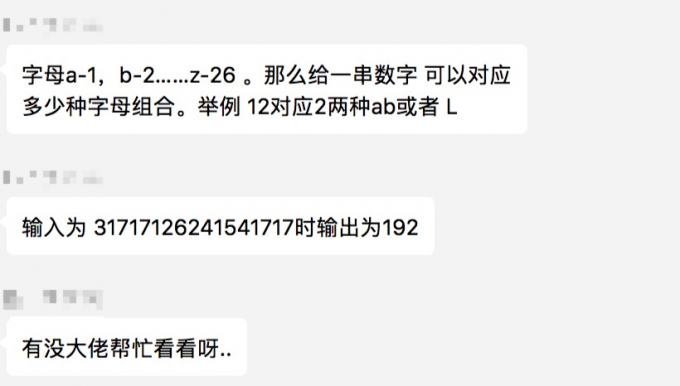Skip to content

# decode-ways``````1. a-z 对应 1-26，不分大小写
2. 111 可以表达多种含义，比如 aaa，ak，ka 等
``````

``````假设输入数字为 S，N 代表了 S 的第 n 项，N-1 代表 S 的第 n-1 项，：

``````

``````def letter_sum(s):
return 0 if len(s) == 0 else get_number(s)

def test_number(s):
return 0 if int(s) == 0 else 1 if int(s) in range(1, 27) else 0

def get_number(s):
if len(s) == 1:
return 1 if test_number(s) else 0
elif len(s) == 2:
return 2 if test_number(s) and test_number(s) else 1
else:
# len(s)>2
if not test_number(s) and test_number(s[:2]):
# just like 011
return get_number(s[2:])
elif test_number(s) and not test_number(s[:2]):
# just like 100，101
return get_number(s[1:])
elif test_number(s) and test_number(s[:2]):
# just like 111
return get_number(s[1:]) + get_number(s[2:])
elif not test_number(s) and not test_number(s[:2]):
# just like 001 011
return 0

print letter_sum('12')
``````

And, It works well~